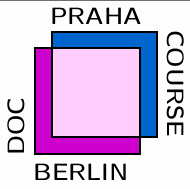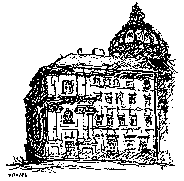# DocCourse, Prague, Jan-Apr 2004This page contains information about my lectures at the doctoral course on Combinatorics, Geometry, and Computation at the Charles University, Prague, January - March 2004.

For more information on the courses in the programme, and how to apply, see the web page (link above).

#### Title

Permutation groups, structures, and polynomials

#### Abstract

The course will begin by discussing the basic concepts of permutation groups, both finite and infinite. Many examples of infinite permutation groups are obtained from homogeneous or omega-categorical structures, and the course will describe some of these. Finite permutation groups occur in enumeration theory; the cycle index of a permutation group has some connections with the Tutte polynomial of a matroid. The theory of species relates these two areas, studying an infinite structure by its finite subsets.

#### Course plan

• Week 1: Permutation groups
• Basic theory of permutation groups
• Orbit-counting and cycle index
• Primitivity, multiple transitivity
• Week 2: Finite permutation groups
• Primitive groups
• O'Nan-Scott theorem
• Applications of CFSG
• Week 3: Homogeneous structures
• The random graph
• The ERS and Fraïssé theorems
• Other homogeneous structures
• Week 4: Counting finite substructures
• Species and their cycle index
• Product, substitution, derivative
• q-analogues
• Week 5: Polynomials and structures
• Codes and matroids
• Weight enumerator and Tutte polynomial
• Cycle index and Tutte polynomial
• Week 6: Revision and exam

Much of the course material can be found in the following sources:
• P. J. Cameron, Permutation Groups, LMS Student Texts 45, Cambridge University Press, Cambridge, 1999.

• P. J. Cameron, The random graph, pp. 331-351 in The Mathematics of Paul Erdös (ed. R. L. Graham and J. Nesetril), Springer, Berlin, 1997.

• P. J. Cameron, Polynomial aspects of codes, matroids and permutation groups, http://www.maths.qmw.ac.uk/~pjc/csgnotes/cmpgpoly.pdf

Background on matroids and species is not specifically required (all that is needed will be covered in the lectures) but can be found in
• J. G. Oxley, Matroid Theory, Oxford University Press, Oxford, 1992.

• F. Bergeron, G. Labelle and P. Leroux, Combinatorial Species and Tree-like Structures, Encyclopedia of Mathematics and its Applications 67, Cambridge University Press, Cambridge, 1998.

Peter J. Cameron (P.J.Cameron@qmul.ac.uk)
2 March 2004